# RD Sharma Solutions for Class 10 Maths Chapter 8 Quadratic Equations Exercise 8.3

A quadratic equation can be solved in many ways. The solution of a quadratic equation by factorization method is the key concept discussed in this exercise. Students facing difficulties in any chapter can access the RD Sharma Solutions Class 10 for free to build up strong knowledge in the subject. The RD Sharma Solutions for Class 10 Maths Chapter 8 Quadratic Equations Exercise 8.3 PDF is also provided below to guide students when solving it.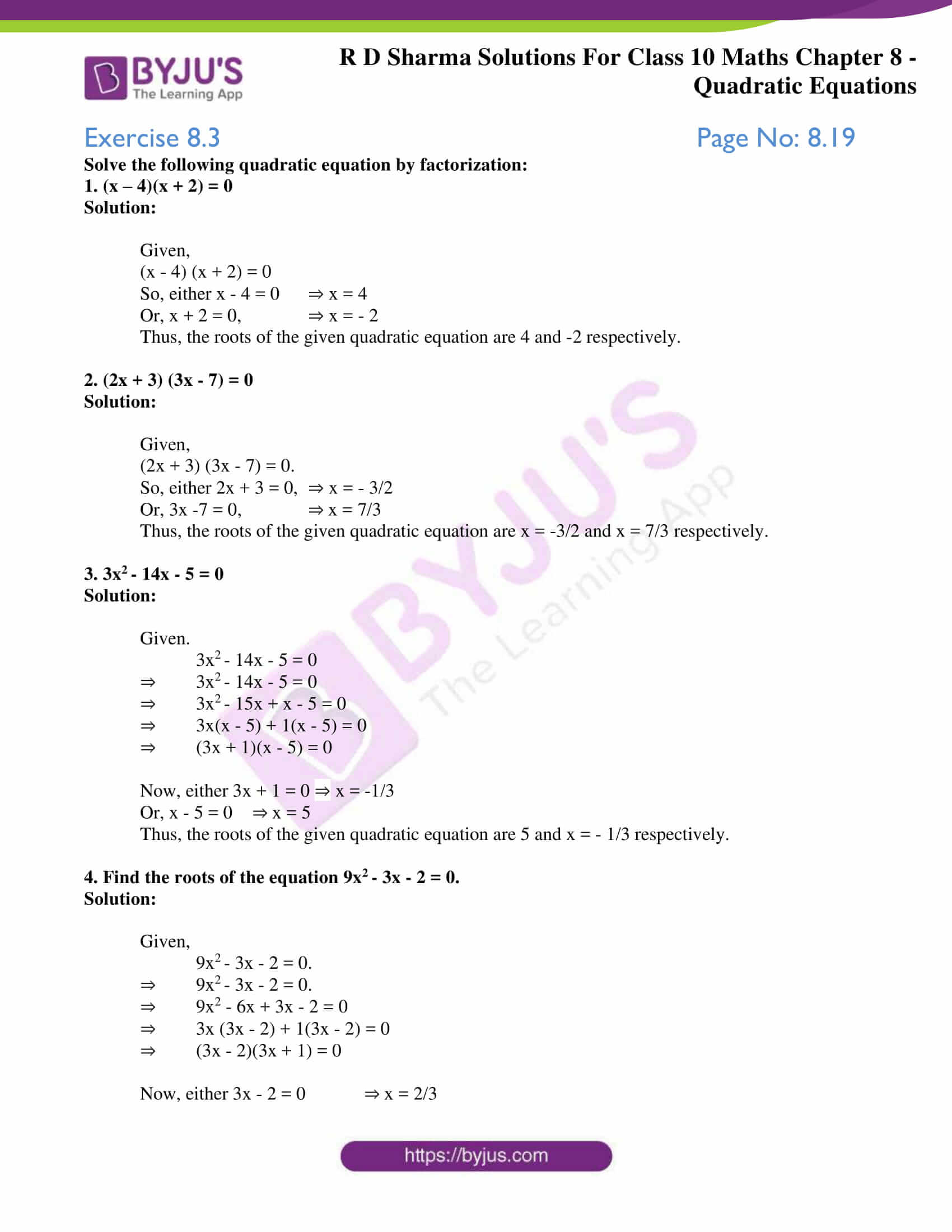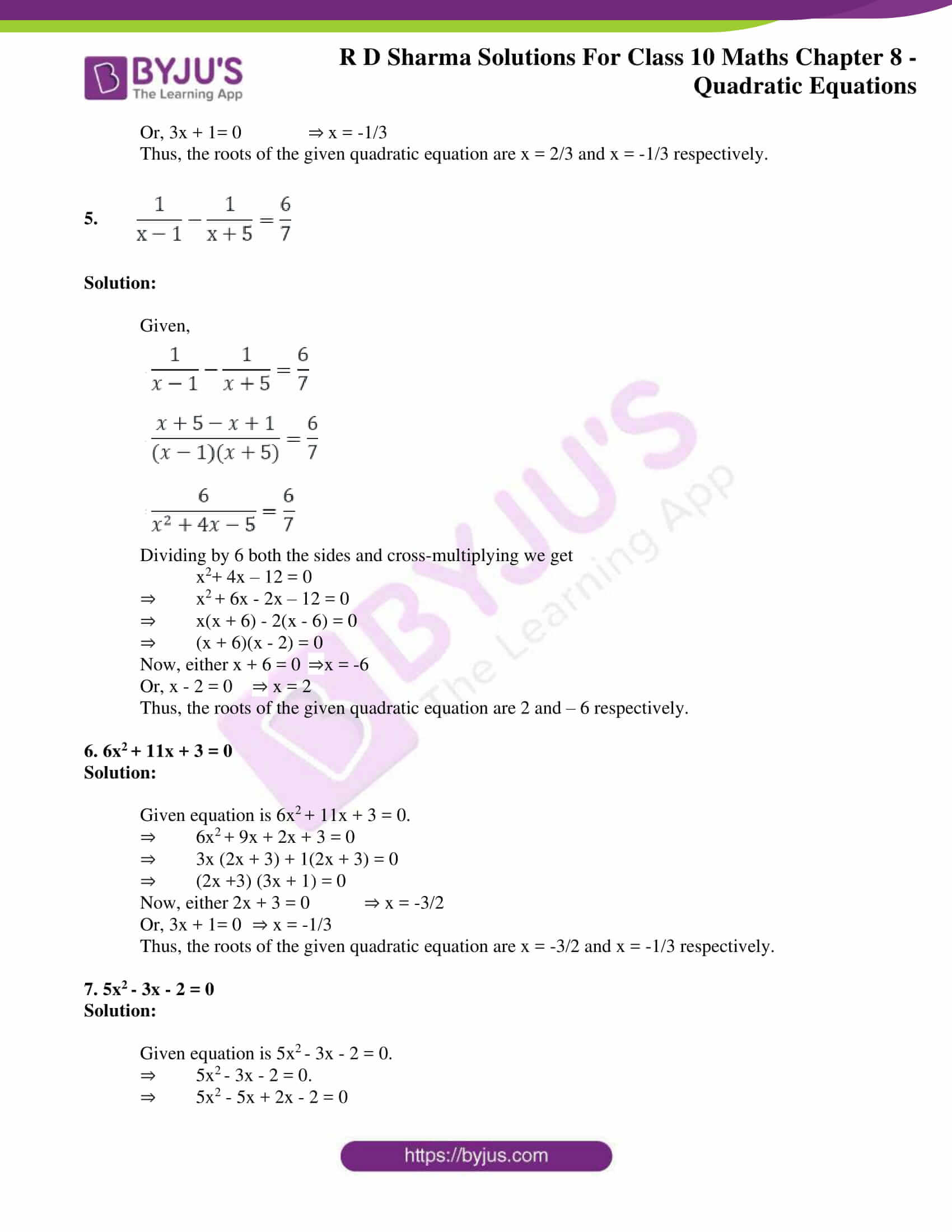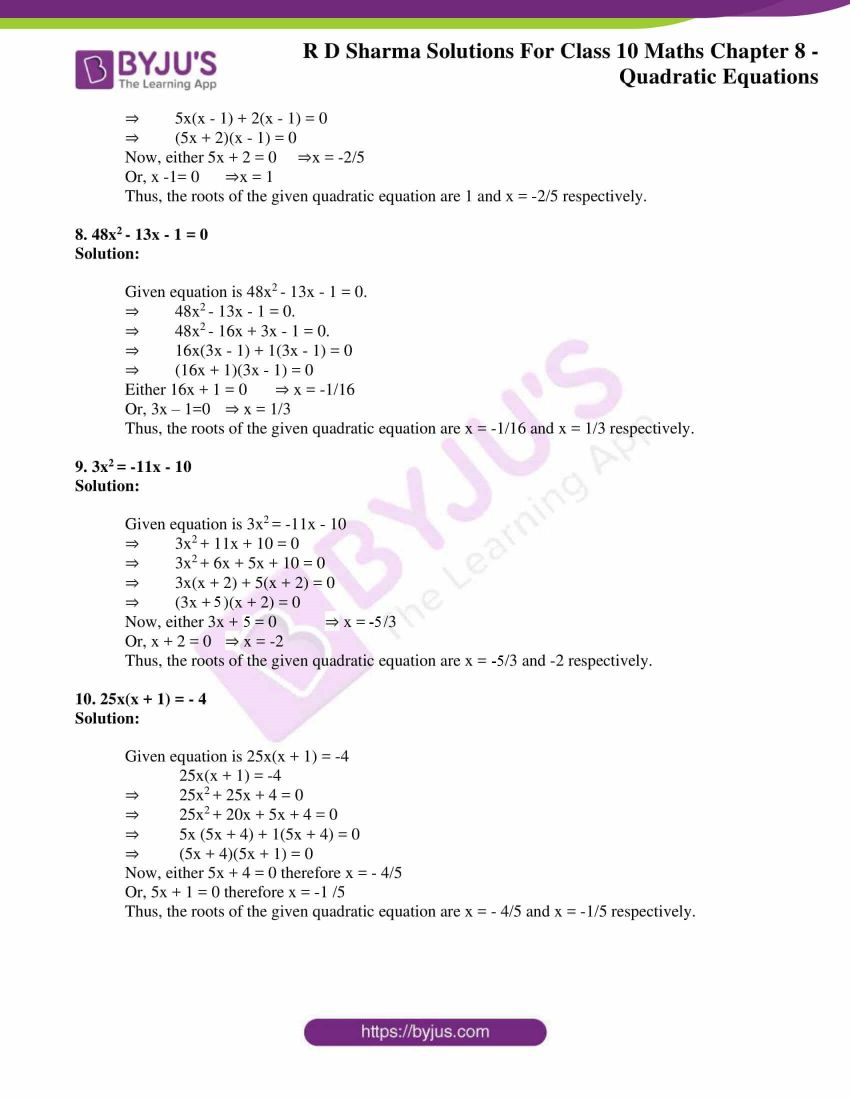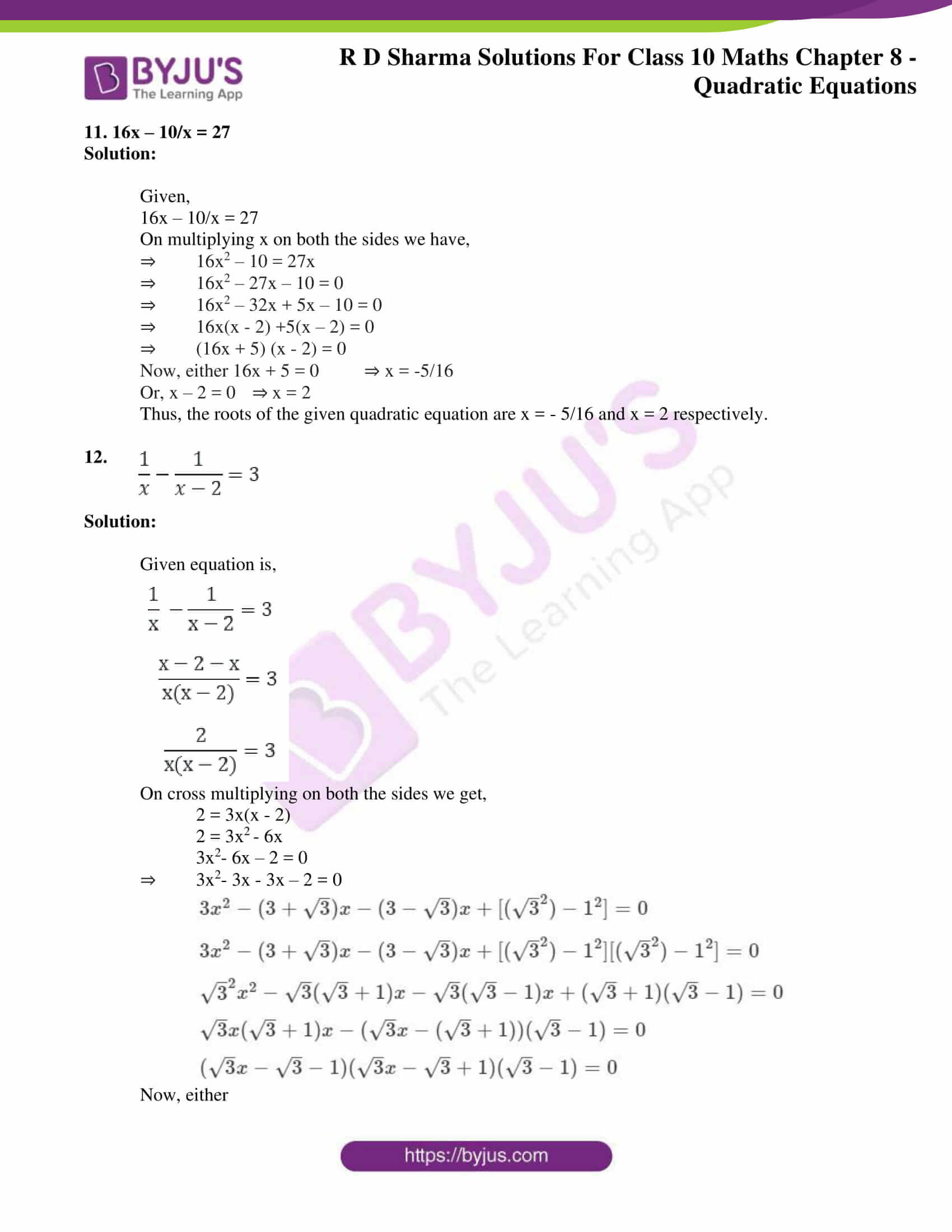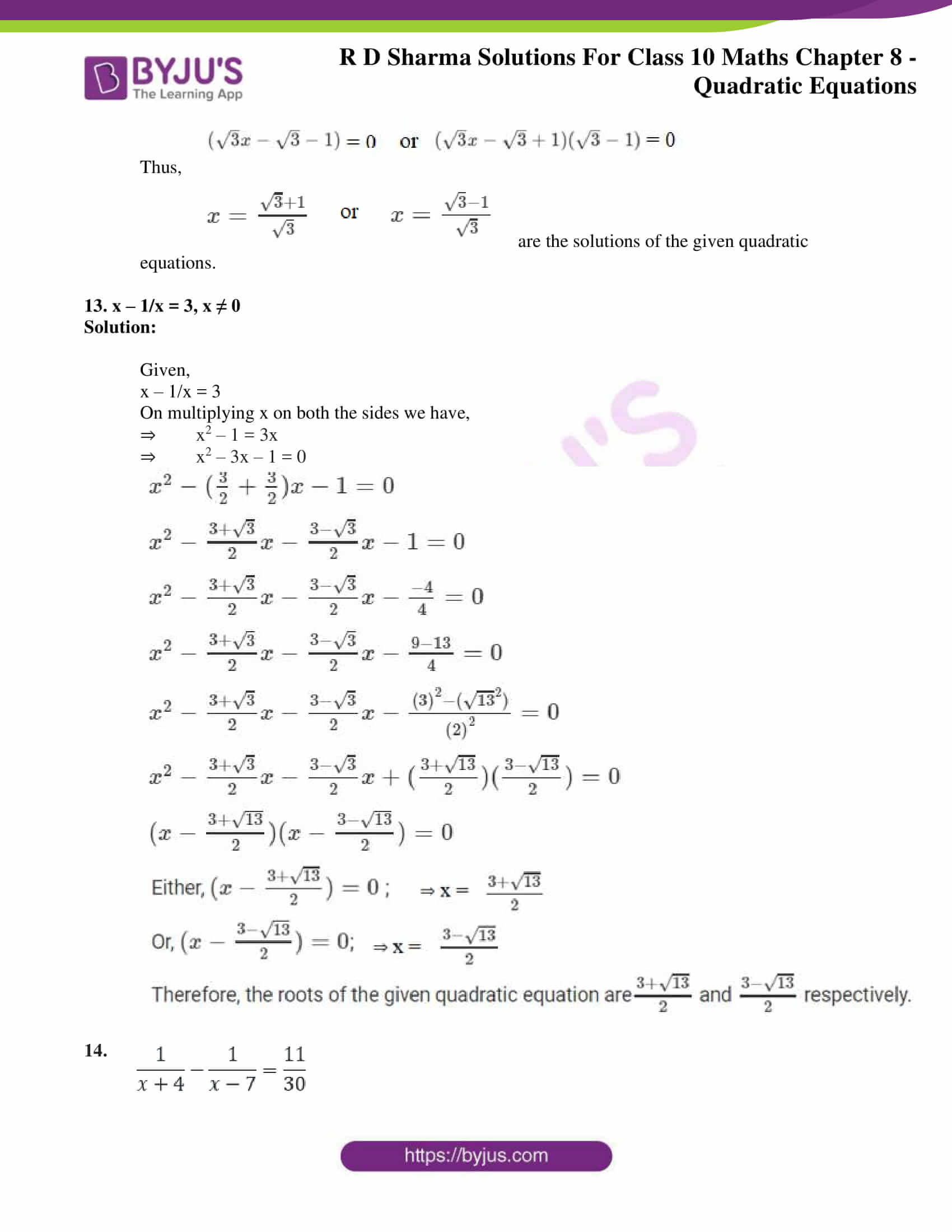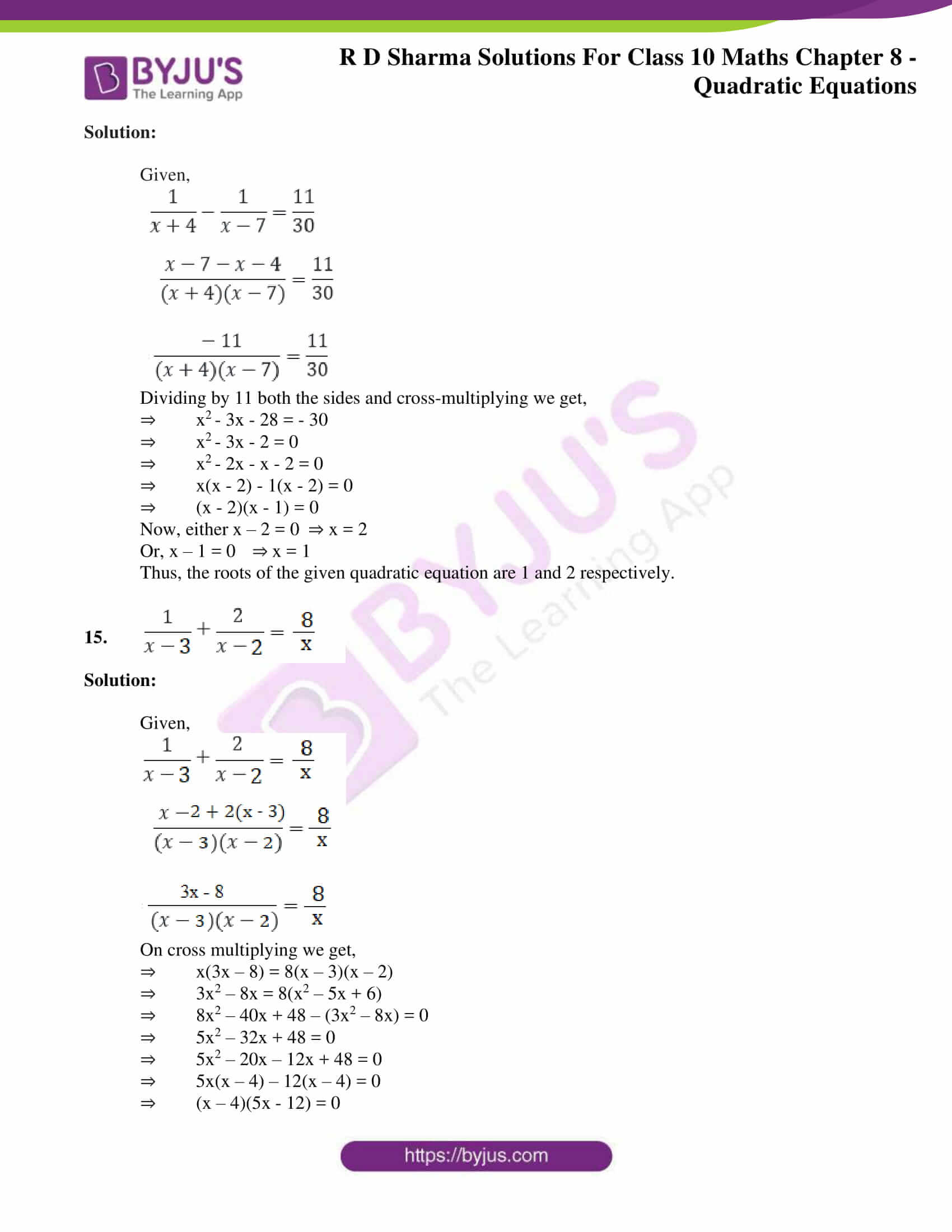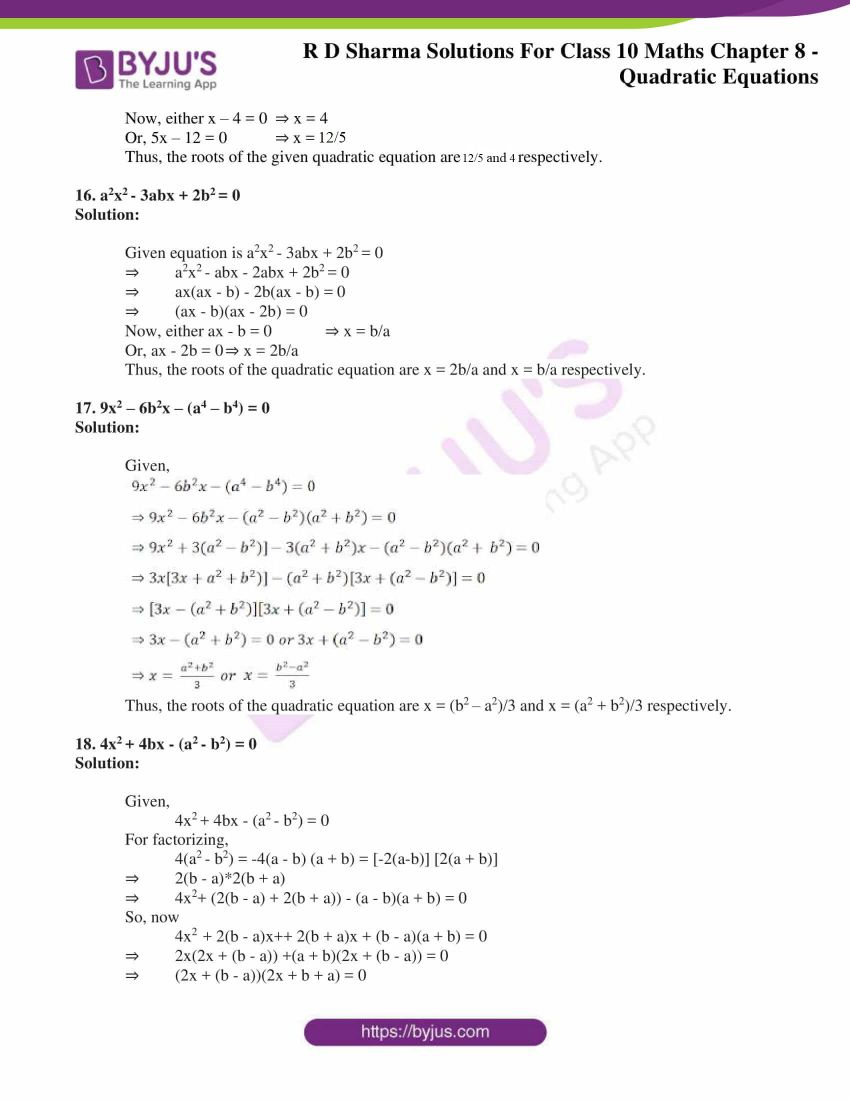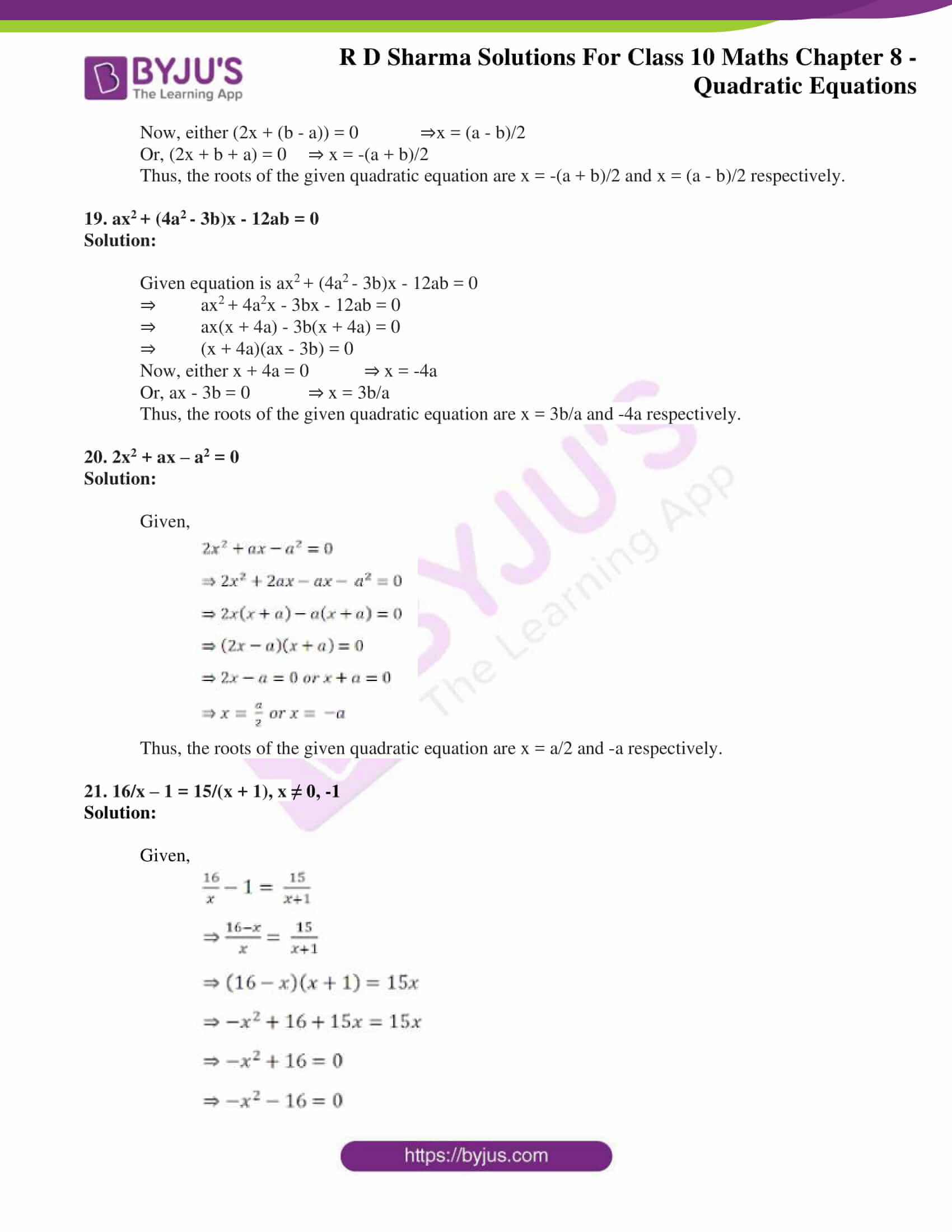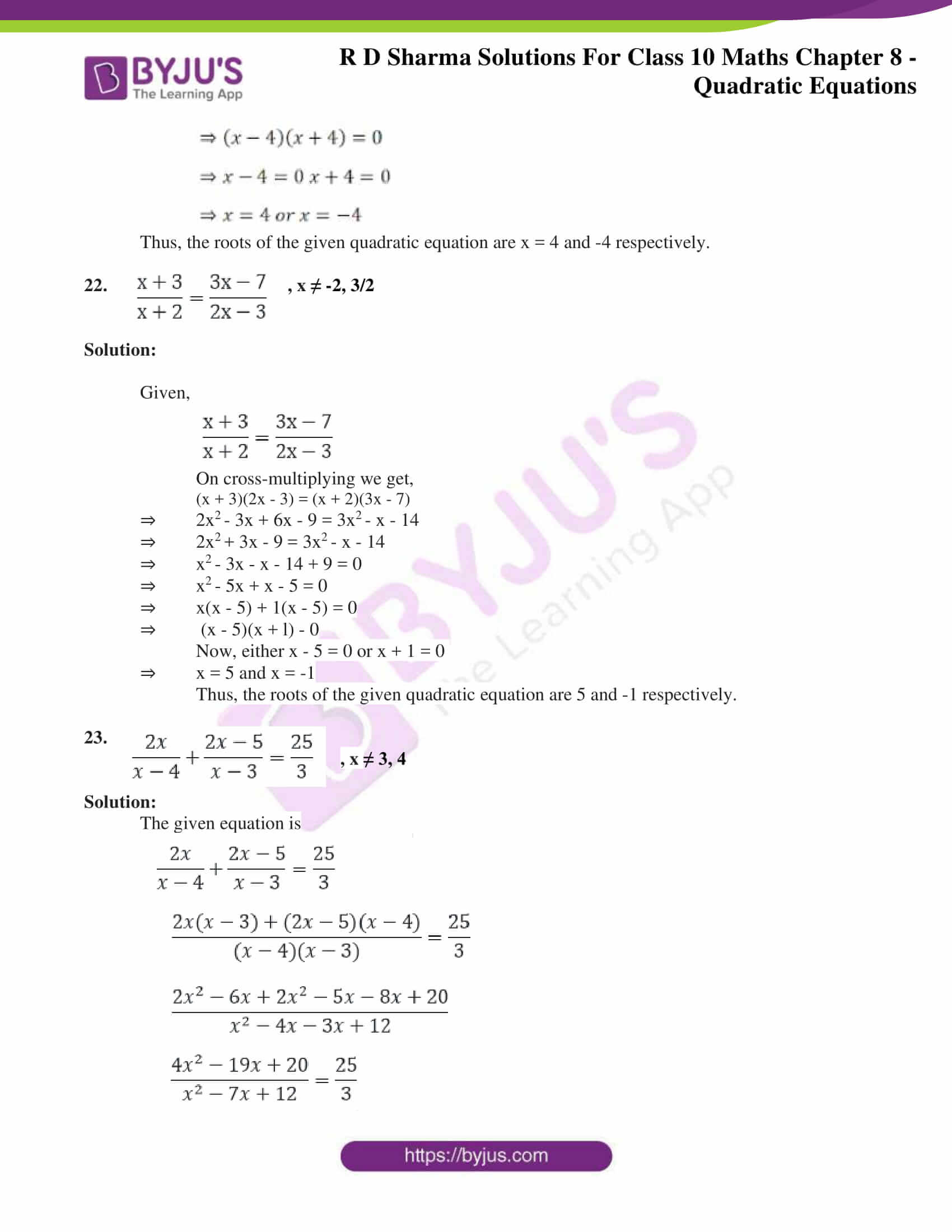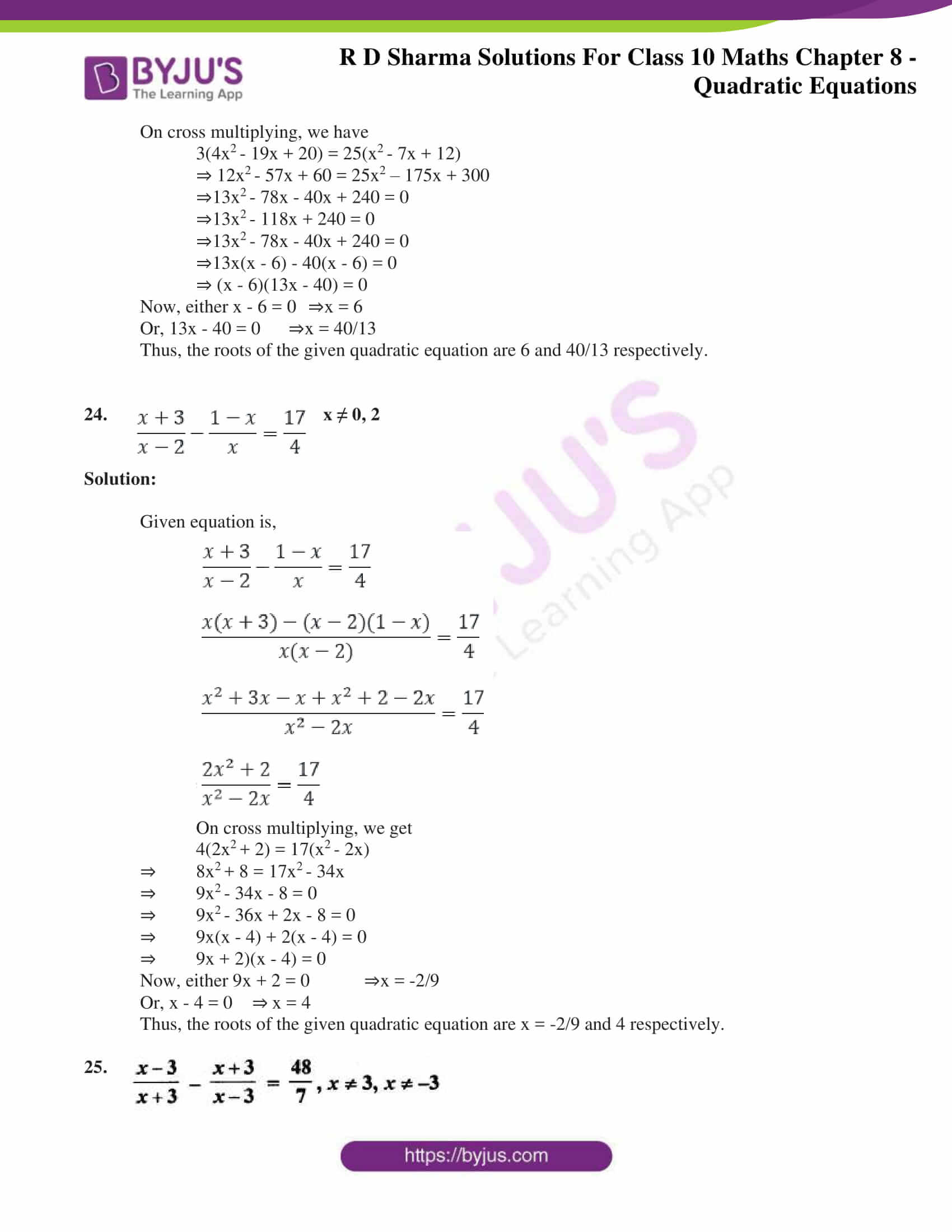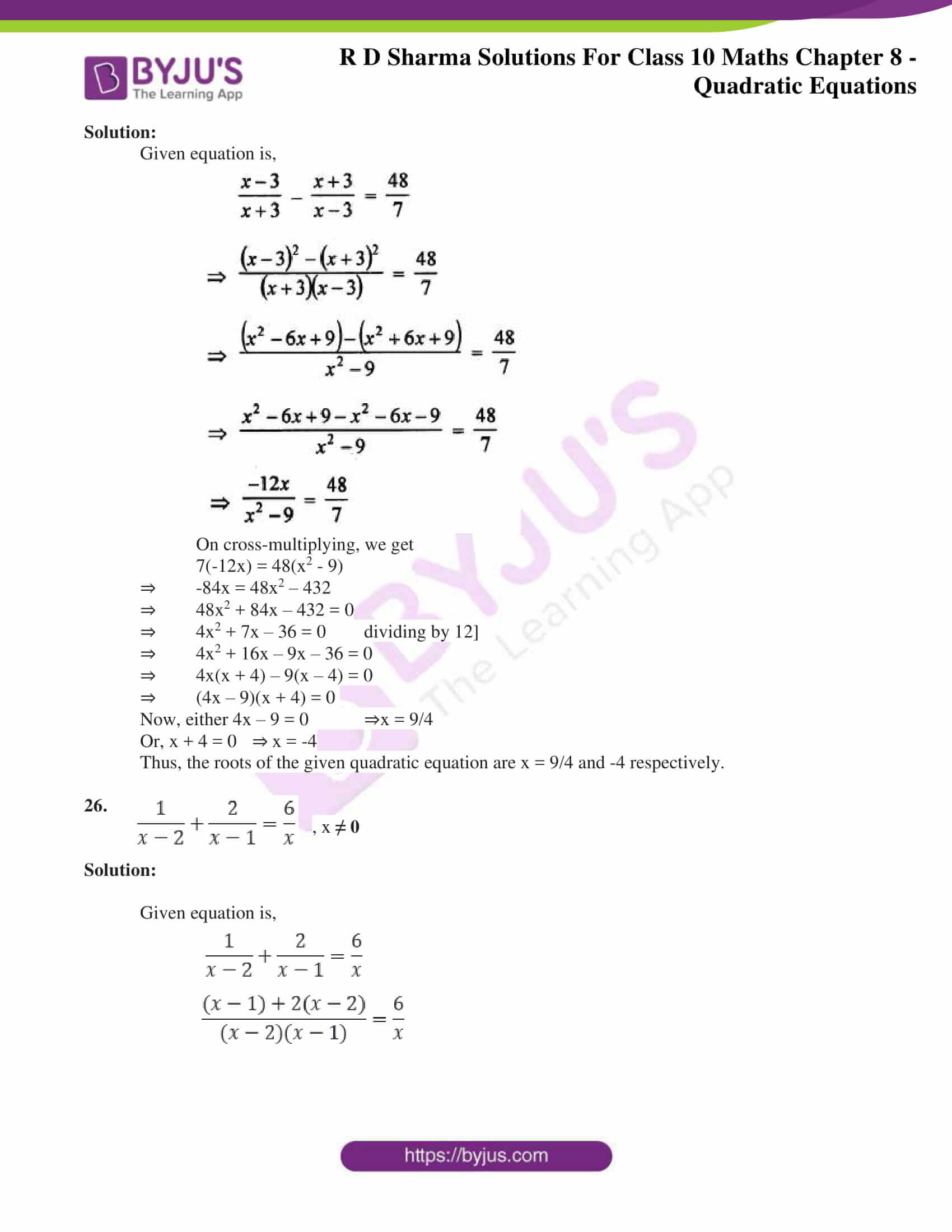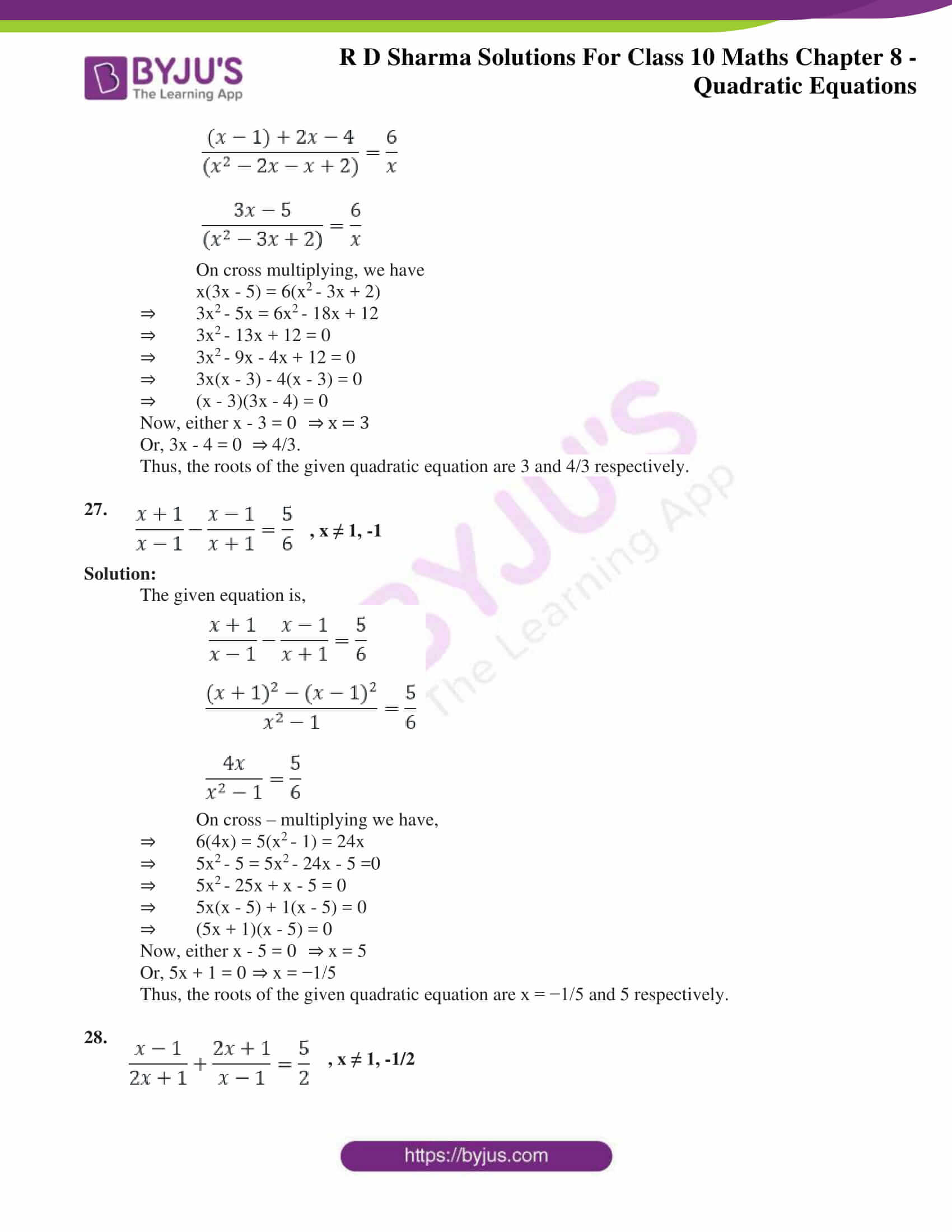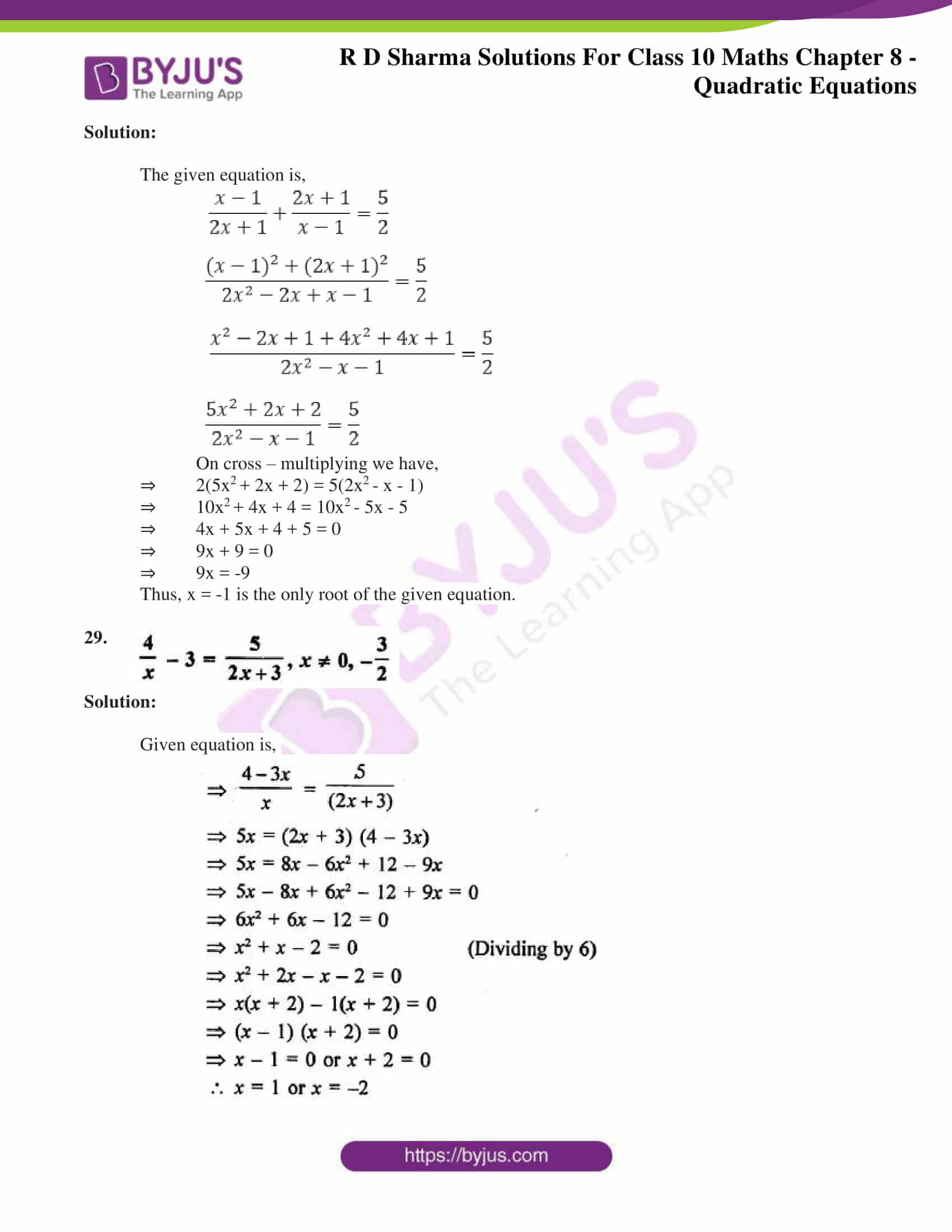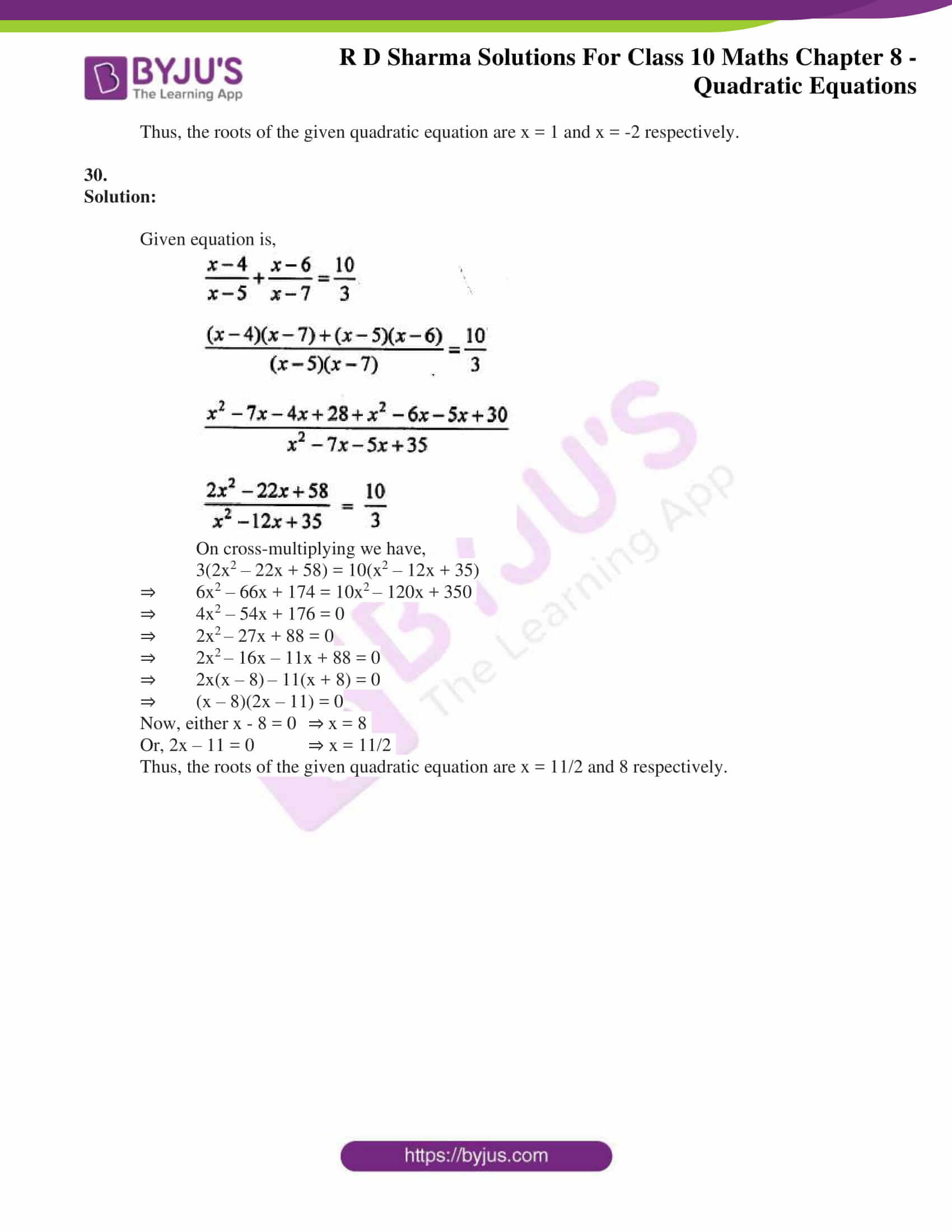### Access RD Sharma Solutions for Class 10 Maths Chapter 8 Quadratic Equations Exercise 8.3

Solve the following quadratic equation by factorization:

1. (x – 4)(x + 2) = 0

Solution:

Given,

(x – 4) (x + 2) = 0

So, either x – 4 = 0 ⇒ x = 4

Or, x + 2 = 0, ⇒ x = – 2

Thus, the roots of the given quadratic equation are 4 and -2 respectively.

2. (2x + 3) (3x – 7) = 0

Solution:

Given,

(2x + 3) (3x – 7) = 0.

So, either 2x + 3 = 0, ⇒ x = – 3/2

Or, 3x -7 = 0, ⇒ x = 7/3

Thus, the roots of the given quadratic equation are x = -3/2 and x = 7/3 respectively.

3. 3x– 14x – 5 = 0

Solution:

Given.

3x– 14x – 5 = 0

⇒ 3x– 14x – 5 = 0

⇒ 3x– 15x + x – 5 = 0

⇒ 3x(x – 5) + 1(x – 5) = 0

⇒ (3x + 1)(x – 5) = 0

Now, either 3x + 1 = 0 ⇒ x = -1/3

Or, x – 5 = 0 ⇒ x = 5

Thus, the roots of the given quadratic equation are 5 and x = – 1/3 respectively.

4. Find the roots of the equation 9x– 3x – 2 = 0.

Solution:

Given,

9x– 3x – 2 = 0.

⇒ 9x– 3x – 2 = 0.

⇒ 9x2 – 6x + 3x – 2 = 0

⇒ 3x (3x – 2) + 1(3x – 2) = 0

⇒ (3x – 2)(3x + 1) = 0

Now, either 3x – 2 = 0 ⇒ x = 2/3

Or, 3x + 1= 0 ⇒ x = -1/3

Thus, the roots of the given quadratic equation are x = 2/3 and x = -1/3 respectively.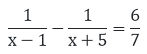5.

Solution:

Given,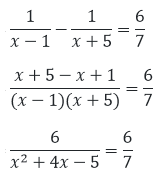Dividing by 6 both the sides and cross-multiplying we get

x2+ 4x – 12 = 0

⇒ x+ 6x – 2x – 12 = 0

⇒ x(x + 6) – 2(x – 6) = 0

⇒ (x + 6)(x – 2) = 0

Now, either x + 6 = 0 ⇒x = -6

Or, x – 2 = 0 ⇒ x = 2

Thus, the roots of the given quadratic equation are 2 and – 6 respectively.

6. 6x+ 11x + 3 = 0

Solution:

Given equation is 6x+ 11x + 3 = 0.

⇒ 6x+ 9x + 2x + 3 = 0

⇒ 3x (2x + 3) + 1(2x + 3) = 0

⇒ (2x +3) (3x + 1) = 0

Now, either 2x + 3 = 0 ⇒ x = -3/2

Or, 3x + 1= 0 ⇒ x = -1/3

Thus, the roots of the given quadratic equation are x = -3/2 and x = -1/3 respectively.

7. 5x– 3x – 2 = 0

Solution:

Given equation is 5x– 3x – 2 = 0.

⇒ 5x– 3x – 2 = 0.

⇒ 5x2 – 5x + 2x – 2 = 0

⇒ 5x(x – 1) + 2(x – 1) = 0

⇒ (5x + 2)(x – 1) = 0

Now, either 5x + 2 = 0 ⇒x = -2/5

Or, x -1= 0 ⇒x = 1

Thus, the roots of the given quadratic equation are 1 and x = -2/5 respectively.

8. 48x– 13x – 1 = 0

Solution:

Given equation is 48x– 13x – 1 = 0.

⇒ 48x– 13x – 1 = 0.

⇒ 48x– 16x + 3x – 1 = 0.

⇒ 16x(3x – 1) + 1(3x – 1) = 0

⇒ (16x + 1)(3x – 1) = 0

Either 16x + 1 = 0 ⇒ x = -1/16

Or, 3x – 1=0 ⇒ x = 1/3

Thus, the roots of the given quadratic equation are x = -1/16 and x = 1/3 respectively.

9. 3x= -11x – 10

Solution:

Given equation is 3x= -11x – 10

⇒ 3x+ 11x + 10 = 0

⇒ 3x+ 6x + 5x + 10 = 0

⇒ 3x(x + 2) + 5(x + 2) = 0

⇒ (3x + 5)(x + 2) = 0

Now, either 3x + 5 = 0 ⇒ x = -5/3

Or, x + 2 = 0 ⇒ x = -2

Thus, the roots of the given quadratic equation are x = -5/3 and -2 respectively.

10. 25x(x + 1) = – 4

Solution:

Given equation is 25x(x + 1) = -4

25x(x + 1) = -4

⇒ 25x+ 25x + 4 = 0

⇒ 25x+ 20x + 5x + 4 = 0

⇒ 5x (5x + 4) + 1(5x + 4) = 0

⇒ (5x + 4)(5x + 1) = 0

Now, either 5x + 4 = 0 therefore x = – 4/5

Or, 5x + 1 = 0 therefore x = -1 /5

Thus, the roots of the given quadratic equation are x = – 4/5 and x = -1/5 respectively.

11. 16x – 10/x = 27

Solution:

Given,

16x – 10/x = 27

On multiplying x on both the sides we have,

⇒ 16x2 – 10 = 27x

⇒ 16x2 – 27x – 10 = 0

⇒ 16x2 – 32x + 5x – 10 = 0

⇒ 16x(x – 2) +5(x – 2) = 0

⇒ (16x + 5) (x – 2) = 0

Now, either 16x + 5 = 0 ⇒ x = -5/16

Or, x – 2 = 0 ⇒ x = 2

Thus, the roots of the given quadratic equation are x = – 5/16 and x = 2 respectively.

12.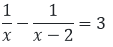Solution:

Given equation is,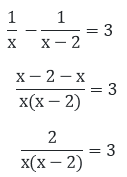On cross multiplying on both the sides we get,

2 = 3x(x – 2)

2 = 3x– 6x

3x2– 6x – 2 = 0

⇒ 3x2– 3x – 3x – 2 = 0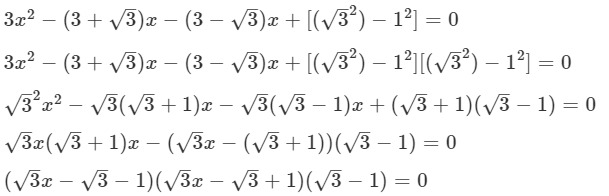Now, either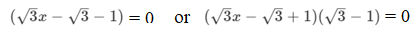Thus,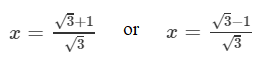are the solutions of the given quadratic equations.

13. x – 1/x = 3, x ≠ 0

Solution:

Given,

x – 1/x = 3

On multiplying x on both the sides we have,

⇒ x2 – 1 = 3x

⇒ x2 – 3x – 1 = 0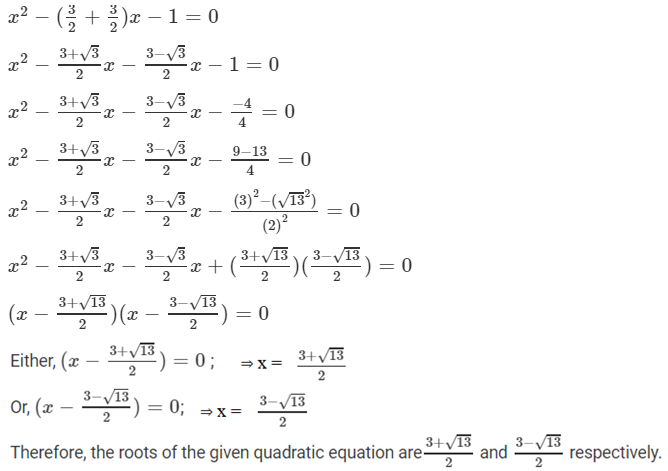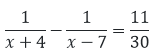14.

Solution:

Given,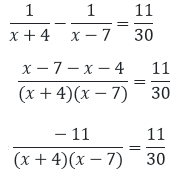Dividing by 11 both the sides and cross-multiplying we get,

⇒ x– 3x – 28 = – 30

⇒ x– 3x – 2 = 0

⇒ x– 2x – x – 2 = 0

⇒ x(x – 2) – 1(x – 2) = 0

⇒ (x – 2)(x – 1) = 0

Now, either x – 2 = 0 ⇒ x = 2

Or, x – 1 = 0 ⇒ x = 1

Thus, the roots of the given quadratic equation are 1 and 2 respectively.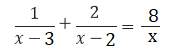15.

Solution:

Given,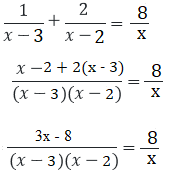On cross multiplying we get,

⇒ x(3x – 8) = 8(x – 3)(x – 2)

⇒ 3x2 – 8x = 8(x2 – 5x + 6)

⇒ 8x2 – 40x + 48 – (3x2 – 8x) = 0

⇒ 5x2 – 32x + 48 = 0

⇒ 5x2 – 20x – 12x + 48 = 0

⇒ 5x(x – 4) – 12(x – 4) = 0

⇒ (x – 4)(5x – 12) = 0

Now, either x – 4 = 0 ⇒ x = 4

Or, 5x – 12 = 0 ⇒ x = 12/5

Thus, the roots of the given quadratic equation are 12/5 and 4 respectively.

16. a2x– 3abx + 2b= 0

Solution:

Given equation is a2x– 3abx + 2b= 0

⇒ a2x– abx – 2abx + 2b= 0

⇒ ax(ax – b) – 2b(ax – b) = 0

⇒ (ax – b)(ax – 2b) = 0

Now, either ax – b = 0 ⇒ x = b/a

Or, ax – 2b = 0 ⇒ x = 2b/a

Thus, the roots of the quadratic equation are x = 2b/a and x = b/a respectively.

17. 9x2 – 6b2x – (a4 – b4) = 0

Solution:

Given,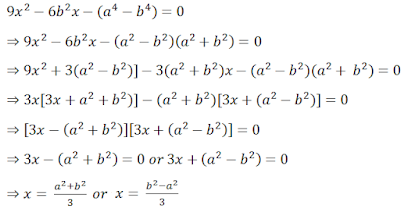Thus, the roots of the quadratic equation are x = (b2 – a2)/3 and x = (a2 + b2)/3 respectively.

18. 4x+ 4bx – (a– b2) = 0

Solution:

Given,

4x+ 4bx – (a– b2) = 0

For factorizing,

4(a– b2) = -4(a – b) (a + b) = [-2(a-b)] [2(a + b)]

⇒ 2(b – a)*2(b + a)

⇒ 4x2+ (2(b – a) + 2(b + a)) – (a – b)(a + b) = 0

So, now

4x2  + 2(b – a)x++ 2(b + a)x + (b – a)(a + b) = 0

⇒ 2x(2x + (b – a)) +(a + b)(2x + (b – a)) = 0

⇒ (2x + (b – a))(2x + b + a) = 0

Now, either (2x + (b – a)) = 0 ⇒x = (a – b)/2

Or, (2x + b + a) = 0 ⇒ x = -(a + b)/2

Thus, the roots of the given quadratic equation are x = -(a + b)/2 and x = (a – b)/2 respectively.

19. ax+ (4a– 3b)x – 12ab = 0

Solution:

Given equation is ax+ (4a– 3b)x – 12ab = 0

⇒ ax+ 4a2x – 3bx – 12ab = 0

⇒ ax(x + 4a) – 3b(x + 4a) = 0

⇒ (x + 4a)(ax – 3b) = 0

Now, either x + 4a = 0 ⇒ x = -4a

Or, ax – 3b = 0 ⇒ x = 3b/a

Thus, the roots of the given quadratic equation are x = 3b/a and -4a respectively.

20. 2x2 + ax – a2 = 0

Solution:

Given,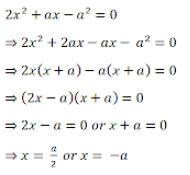Thus, the roots of the given quadratic equation are x = a/2 and -a respectively.

21. 16/x – 1 = 15/(x + 1), x ≠ 0, -1

Solution:

Given,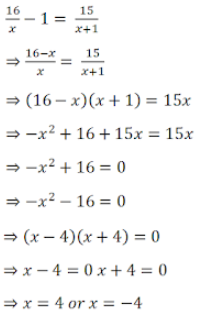Thus, the roots of the given quadratic equation are x = 4 and -4 respectively.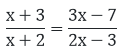22. , x ≠ -2, 3/2

Solution:

Given,On cross-multiplying we get,

(x + 3)(2x – 3) = (x + 2)(3x – 7)

⇒ 2x– 3x + 6x – 9 = 3x– x – 14

⇒ 2x+ 3x – 9 = 3x– x – 14

⇒ x– 3x – x – 14 + 9 = 0

⇒ x– 5x + x – 5 = 0

⇒ x(x – 5) + 1(x – 5) = 0

⇒ (x – 5)(x + l) – 0

Now, either x – 5 = 0 or x + 1 = 0

⇒ x = 5 and x = -1

Thus, the roots of the given quadratic equation are 5 and -1 respectively.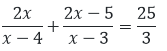23.

, x ≠ 3, 4

Solution:

The given equation is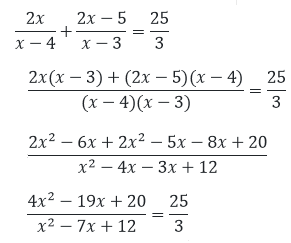On cross multiplying, we have

3(4x– 19x + 20) = 25(x– 7x + 12)

⇒ 12x– 57x + 60 = 25x2 – 175x + 300

⇒13x– 78x – 40x + 240 = 0

⇒13x– 118x + 240 = 0

⇒13x– 78x – 40x + 240 = 0

⇒13x(x – 6) – 40(x – 6) = 0

⇒ (x – 6)(13x – 40) = 0

Now, either x – 6 = 0 ⇒x = 6

Or, 13x – 40 = 0 ⇒x = 40/13

Thus, the roots of the given quadratic equation are 6 and 40/13 respectively.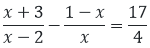24. x ≠ 0, 2

Solution:

Given equation is,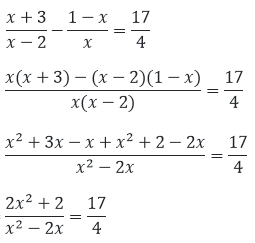On cross multiplying, we get

4(2x+ 2) = 17(x– 2x)

⇒ 8x+ 8 = 17x– 34x

⇒ 9x– 34x – 8 = 0

⇒ 9x– 36x + 2x – 8 = 0

⇒ 9x(x – 4) + 2(x – 4) = 0

⇒ 9x + 2)(x – 4) = 0

Now, either 9x + 2 = 0 ⇒x = -2/9

Or, x – 4 = 0 ⇒ x = 4

Thus, the roots of the given quadratic equation are x = -2/9 and 4 respectively.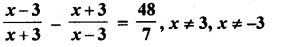25.

Solution:

Given equation is,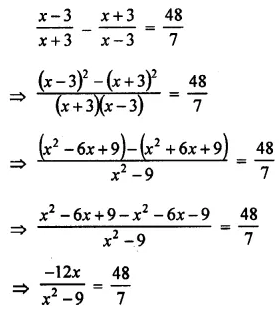On cross-multiplying, we get

7(-12x) = 48(x2 – 9)

⇒ -84x = 48x2 – 432

⇒ 48x2 + 84x – 432 = 0

⇒ 4x2 + 7x – 36 = 0 dividing by 12]

⇒ 4x2 + 16x – 9x – 36 = 0

⇒ 4x(x + 4) – 9(x – 4) = 0

⇒ (4x – 9)(x + 4) = 0

Now, either 4x – 9 = 0 ⇒x = 9/4

Or, x + 4 = 0 ⇒ x = -4

Thus, the roots of the given quadratic equation are x = 9/4 and -4 respectively.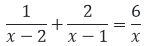26.

, x ≠ 0

Solution:

Given equation is,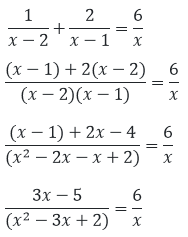On cross multiplying, we have

x(3x – 5) = 6(x– 3x + 2)

⇒ 3x– 5x = 6x– 18x + 12

⇒ 3x– 13x + 12 = 0

⇒ 3x– 9x – 4x + 12 = 0

⇒ 3x(x – 3) – 4(x – 3) = 0

⇒ (x – 3)(3x – 4) = 0

Now, either x – 3 = 0 ⇒ x = 3

Or, 3x – 4 = 0 ⇒ 4/3.

Thus, the roots of the given quadratic equation are 3 and 4/3 respectively.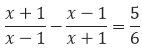27.

, x ≠ 1, -1

Solution:

The given equation is,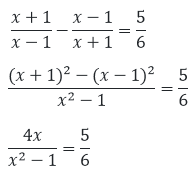On cross – multiplying we have,

⇒ 6(4x) = 5(x– 1) = 24x

⇒ 5x– 5 = 5x– 24x – 5 =0

⇒ 5x– 25x + x – 5 = 0

⇒ 5x(x – 5) + 1(x – 5) = 0

⇒ (5x + 1)(x – 5) = 0

Now, either x – 5 = 0 ⇒ x = 5

Or, 5x + 1 = 0 ⇒ x = −1/5

Thus, the roots of the given quadratic equation are x = −1/5 and 5 respectively.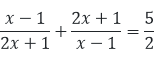28.

, x ≠ 1, -1/2

Solution:

The given equation is,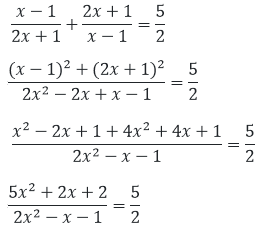On cross – multiplying we have,

⇒ 2(5x+ 2x + 2) = 5(2x– x – 1)

⇒ 10x+ 4x + 4 = 10x– 5x – 5

⇒ 4x + 5x + 4 + 5 = 0

⇒ 9x + 9 = 0

⇒ 9x = -9

Thus, x = -1 is the only root of the given equation.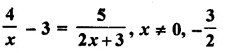29.

Solution:

Given equation is,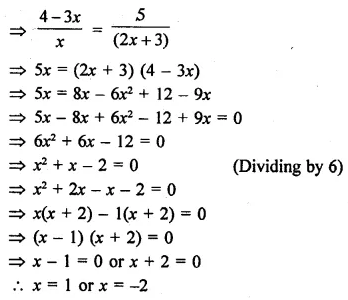Thus, the roots of the given quadratic equation are x = 1 and x = -2 respectively.

30.

Solution:

Given equation is,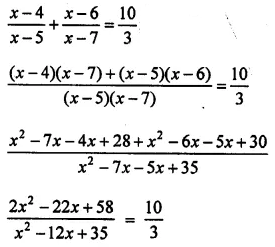On cross-multiplying we have,

3(2x2 – 22x + 58) = 10(x2 – 12x + 35)

⇒ 6x2 – 66x + 174 = 10x2 – 120x + 350

⇒ 4x2 – 54x + 176 = 0

⇒ 2x2 – 27x + 88 = 0

⇒ 2x2 – 16x – 11x + 88 = 0

⇒ 2x(x – 8) – 11(x + 8) = 0

⇒ (x – 8)(2x – 11) = 0

Now, either x – 8 = 0 ⇒ x = 8

Or, 2x – 11 = 0 ⇒ x = 11/2

Thus, the roots of the given quadratic equation are x = 11/2 and 8 respectively.